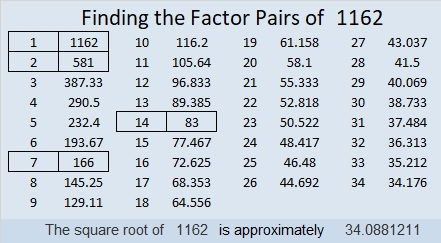# 1162 Is a Pentagonal Number

1162 is the 28th pentagonal number because (3(28²) – 28)/2 = 1162. I created the graphic below so you can see all the pentagonal numbers up to 1162 and figure out why all of them are called pentagonal numbers.Here’s more about the number 1162:

• 1162 is a composite number.
• Prime factorization: 1162 = 2 × 7 × 83
• The exponents in the prime factorization are 1, 1, and 1. Adding one to each and multiplying we get (1 + 1)(1 + 1)(1 + 1) = 2 × 2 × 2 = 8. Therefore 1162 has exactly 8 factors.
• Factors of 1162: 1, 2, 7, 14, 83, 166, 581, 1162
• Factor pairs: 1162 = 1 × 1162, 2 × 581, 7 × 166, or 14 × 83
• 1162 has no square factors that allow its square root to be simplified. √1162 ≈ 34.088121162 is a palindrome in three bases:
It’s 343 in BASE 19 because 3(19²) + 4(19) + 3(1) = 1162,
2I2 in BASE 20 (I is 18 base 10) because 2(20²) + 18(20) + 2(1) = 1162, and
1G1 in BASE 27 (G is 16 base 10) because 27² + 16(27) + 1 = 1162